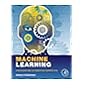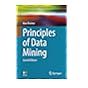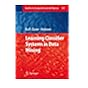Normal view MARC view ISBD view

# Machine learning: a Bayesian and optimization perspective

Publisher: Amsterdam Academic Press 2015Description: xxi, 1050 p.ISBN: 9780128015223.DDC classification: 006.31 Summary: This tutorial text gives a unifying perspective on machine learning by covering both probabilistic and deterministic approaches -which are based on optimization techniques – together with the Bayesian inference approach, whose essence lies in the use of a hierarchy of probabilistic models. The book presents the major machine learning methods as they have been developed in different disciplines, such as statistics, statistical and adaptive signal processing and computer science. Focusing on the physical reasoning behind the mathematics, all the various methods and techniques are explained in depth, supported by examples and problems, giving an invaluable resource to the student and researcher for understanding and applying machine learning concepts. The book builds carefully from the basic classical methods to the most recent trends, with chapters written to be as self-contained as possible, making the text suitable for different courses: pattern recognition, statistical/adaptive signal processing, statistical/Bayesian learning, as well as short courses on sparse modeling, deep learning, and probabilistic graphical models. (http://store.elsevier.com/Machine-Learning/Sergios-Theodoridis/isbn-9780128017227/)
Tags from this library: No tags from this library for this title.
average rating: 0.0 (0 votes)
Item type Current location Item location Collection Call number Status Date due Barcode
Books Vikram Sarabhai Library
Slot 104 (0 Floor, West Wing) Non-fiction 006.31 T4M2 (Browse shelf) Available 192563
##### Browsing Vikram Sarabhai Library Shelves , Collection code: Non-fiction Close shelf browser006.31 T4M2 Machine learning: a Bayesian and optimization perspective 006.31 Z4E6 Ensemble methods: foundations and algorithms 006.312 B7P7-2013 Principles of data mining 006.312 B8L3 Learning classifier systems in data mining

Chapter 1: Introduction

Abstract
1.1 What Machine Learning is About
1.2 Structure and a Road Map of the Book

Chapter 2: Probability and Stochastic Processes

Abstract
2.1 Introduction
2.2 Probability and Random Variables
2.3 Examples of Distributions
2.4 Stochastic Processes
2.5 Information Theory
2.6 Stochastic Convergence
Problems

Chapter 3: Learning in Parametric Modeling: Basic Concepts and Directions

Abstract
3.1 Introduction
3.2 Parameter Estimation: The Deterministic Point of View
3.3 Linear Regression
3.4 Classification
3.5 Biased Versus Unbiased Estimation
3.6 The Cramér-Rao Lower Bound
3.7 Sufficient Statistic
3.8 Regularization
3.9 The Bias-Variance Dilemma
3.10 Maximum Likelihood Method
3.11 Bayesian Inference
3.12 Curse of Dimensionality
3.13 Validation
3.14 Expected and Empirical Loss Functions
3.15 Nonparametric Modeling and Estimation
Problems

Chapter 4: Mean-Square Error Linear Estimation

Abstract
4.1 Introduction
4.2 Mean-Square Error Linear Estimation: The Normal Equations

Chapter 5: Stochastic Gradient Descent: The LMS Algorithm and its Family

Abstract
5.1 Introduction
5.2 The Steepest Descent Method
5.3 Application to the Mean-Square Error Cost Function
5.4 Stochastic Approximation
5.5 The Least-Mean-Squares Adaptive Algorithm
5.6 The Affine Projection Algorithm
5.7 The Complex-Valued Case
5.8 Relatives of the LMS
5.9 Simulation Examples
5.10 Adaptive Decision Feedback Equalization
5.11 The Linearly Constrained LMS
5.12 Tracking Performance of the LMS in Nonstationary Environments
5.13 Distributed Learning: The Distributed LMS
5.14 A Case Study: Target Localization
5.15 Some Concluding Remarks: Consensus Matrix
Problems
MATLAB Exercises

Chapter 6: The Least-Squares Family

Abstract
6.1 Introduction
6.2 Least-Squares Linear Regression: A Geometric Perspective
6.3 Statistical Properties of the LS Estimator
6.4 Orthogonalizing the Column Space of X: The SVD Method
6.5 Ridge Regression
6.6 The Recursive Least-Squares Algorithm
6.7 Newton’s Iterative Minimization Method
6.8 Steady-State Performance of the RLS
6.9 Complex-Valued Data: The Widely Linear RLS
6.10 Computational Aspects of the LS Solution
6.11 The Coordinate and Cyclic Coordinate Descent Methods
6.12 Simulation Examples
6.13 Total-Least-Squares
Problems

Chapter 7: Classification: A Tour of the Classics

Abstract
7.1 Introduction
7.2 Bayesian Classification
7.3 Decision (Hyper)Surfaces
7.4 The Naive Bayes Classifier
7.5 The Nearest Neighbor Rule
7.6 Logistic Regression
7.7 Fisher’s Linear Discriminant
7.8 Classification Trees
7.9 Combining Classifiers
7.10 The Boosting Approach
7.11 Boosting Trees
7.12 A Case Study: Protein Folding Prediction
Problems

Chapter 8: Parameter Learning: A Convex Analytic Path

Abstract
8.1 Introduction
8.2 Convex Sets and Functions
8.3 Projections onto Convex Sets
8.4 Fundamental Theorem of Projections onto Convex Sets
8.5 A Parallel Version of POCS
8.6 From Convex Sets to Parameter Estimation and Machine Learning
8.7 Infinite Many Closed Convex Sets: The Online Learning Case
8.8 Constrained Learning
8.9 The Distributed APSM
8.10 Optimizing Nonsmooth Convex Cost Functions
8.11 Regret Analysis
8.12 Online Learning and Big Data Applications: A Discussion
8.13 Proximal Operators
8.14 Proximal Splitting Methods for Optimization
Problems
MATLAB Exercises
8.15 Appendix to Chapter 8

Chapter 9: Sparsity-Aware Learning: Concepts and Theoretical Foundations

Abstract
9.1 Introduction
9.2 Searching for a Norm
9.3 The Least Absolute Shrinkage and Selection Operator (LASSO)
9.4 Sparse Signal Representation
9.5 In Search of the Sparsest Solution
9.6 Uniqueness of the ℓ0 Minimizer
9.7 Equivalence of ℓ0 and ℓ1 Minimizers: Sufficiency Conditions
9.8 Robust Sparse Signal Recovery from Noisy Measurements
9.9 Compressed Sensing: The Glory of Randomness
9.10 A Case Study: Image De-Noising
Problems

Chapter 10: Sparsity-Aware Learning: Algorithms and Applications

Abstract
10.1 Introduction
10.2 Sparsity-Promoting Algorithms
10.3 Variations on the Sparsity-Aware Theme
10.4 Online Sparsity-Promoting Algorithms
10.5 Learning Sparse Analysis Models
10.6 A Case Study: Time-Frequency Analysis
10.7 Appendix to Chapter 10: Some Hints from the Theory of Frames
Problems

Chapter 11: Learning in Reproducing Kernel Hilbert Spaces

Abstract
11.1 Introduction
11.2 Generalized Linear Models
11.3 Volterra, Wiener, and Hammerstein Models
11.4 Cover’s Theorem: Capacity of a Space in Linear Dichotomies
11.5 Reproducing Kernel Hilbert Spaces
11.6 Representer Theorem
11.7 Kernel Ridge Regression
11.8 Support Vector Regression
11.9 Kernel Ridge Regression Revisited
11.10 Optimal Margin Classification: Support Vector Machines
11.11 Computational Considerations
11.12 Online Learning in RKHS
11.13 Multiple Kernel Learning
11.14 Nonparametric Sparsity-Aware Learning: Additive Models
11.15 A Case Study: Authorship Identification
Problems

Chapter 12: Bayesian Learning: Inference and the EM Algorithm

Abstract
12.1 Introduction
12.2 Regression: A Bayesian Perspective
12.3 The Evidence Function and Occam’s Razor Rule
12.4 Exponential Family of Probability Distributions
12.5 Latent Variables and the EM Algorithm
12.6 Linear Regression and the EM Algorithm
12.7 Gaussian Mixture Models
12.8 Combining Learning Models: A Probabilistic Point of View
Problems
MATLAB Exercises
12.9 Appendix to Chapter 12

Chapter 13: Bayesian Learning: Approximate Inference and Nonparametric Models

Abstract
13.1 Introduction
13.2 Variational Approximation in Bayesian Learning
13.3 A Variational Bayesian Approach to Linear Regression
13.4 A Variational Bayesian Approach to Gaussian Mixture Modeling
13.5 When Bayesian Inference Meets Sparsity
13.6 Sparse Bayesian Learning (SBL)
13.7 The Relevance Vector Machine Framework
13.8 Convex Duality and Variational Bounds
13.9 Sparsity-Aware Regression: A Variational Bound Bayesian Path
13.10 Sparsity-Aware Learning: Some Concluding Remarks
13.11 Expectation Propagation
13.12 Nonparametric Bayesian Modeling
13.13 Gaussian Processes
13.14 A Case Study: Hyperspectral Image Unmixing
Problems

Chapter 14: Monte Carlo Methods

Abstract
14.1 Introduction
14.2 Monte Carlo Methods: The Main Concept
14.3 Random Sampling Based on Function Transformation
14.4 Rejection Sampling
14.5 Importance Sampling
14.6 Monte Carlo Methods and the EM Algorithm
14.7 Markov Chain Monte Carlo Methods
14.8 The Metropolis Method
14.9 Gibbs Sampling
14.10 In Search of More Efficient Methods: A Discussion
14.11 A Case Study: Change-Point Detection
Problems

Chapter 15: Probabilistic Graphical Models: Part I

Abstract
15.1 Introduction
15.2 The Need for Graphical Models
15.3 Bayesian Networks and the Markov Condition
15.4 Undirected Graphical Models
15.5 Factor Graphs
15.6 Moralization of Directed Graphs
15.7 Exact Inference Methods: Message-Passing Algorithms
Problems

Chapter 16: Probabilistic Graphical Models: Part II

Abstract
16.1 Introduction
16.2 Triangulated Graphs and Junction Trees
16.3 Approximate Inference Methods
16.4 Dynamic Graphical Models
16.5 Hidden Markov Models
16.6 Beyond HMMs: A Discussion
16.7 Learning Graphical Models
Problems

Chapter 17: Particle Filtering

Abstract
17.1 Introduction
17.2 Sequential Importance Sampling
17.3 Kalman and Particle Filtering
17.4 Particle Filtering
Problems

Chapter 18: Neural Networks and Deep Learning

Abstract
18.1 Introduction
18.2 The Perceptron
18.3 Feed-Forward Multilayer Neural Networks
18.4 The Backpropagation Algorithm
18.5 Pruning the Network
18.6 Universal Approximation Property of Feed-Forward Neural Networks
18.7 Neural Networks: A Bayesian Flavor
18.8 Learning Deep Networks
18.9 Deep Belief Networks
18.10 Variations on the Deep Learning Theme
18.11 Case Study: A Deep Network for Optical Character Recognition
18.12 CASE Study: A Deep Autoencoder
18.13 Example: Generating Data via a DBN
Problems
MATLAB Exercises

Chapter 19: Dimensionality Reduction and Latent Variables Modeling

Abstract
19.1 Introduction
19.2 Intrinsic Dimensionality
19.3 Principle Component Analysis
19.4 Canonical Correlation Analysis
19.5 Independent Component Analysis
19.6 Dictionary Learning: The k-SVD Algorithm
19.7 Nonnegative Matrix Factorization
19.8 Learning Low-Dimensional Models: A Probabilistic Perspective
19.9 Nonlinear Dimensionality Reduction
19.10 Low-Rank Matrix Factorization: A Sparse Modeling Path
19.11 A Case Study: fMRI Data Analysis
Problems

Appendix A: Linear Algebra
A.1 Properties of Matrices
A.2 Positive Definite and Symmetric Matrices
A.3 Wirtinger Calculus

Appendix B: Probability Theory and Statistics
B.1 Cramér-Rao Bound
B.2 Characteristic Functions
B.3 Moments and Cumulants
B.4 Edgeworth Expansion of a pdf

Appendix C: Hints on Constrained Optimization
C.1 Equality Constraints
C.2 Inequality Constrains

Index

This tutorial text gives a unifying perspective on machine learning by covering both probabilistic and deterministic approaches -which are based on optimization techniques – together with the Bayesian inference approach, whose essence lies in the use of a hierarchy of probabilistic models.

The book presents the major machine learning methods as they have been developed in different disciplines, such as statistics, statistical and adaptive signal processing and computer science. Focusing on the physical reasoning behind the mathematics, all the various methods and techniques are explained in depth, supported by examples and problems, giving an invaluable resource to the student and researcher for understanding and applying machine learning concepts.

The book builds carefully from the basic classical methods to the most recent trends, with chapters written to be as self-contained as possible, making the text suitable for different courses: pattern recognition, statistical/adaptive signal processing, statistical/Bayesian learning, as well as short courses on sparse modeling, deep learning, and probabilistic graphical models.

(http://store.elsevier.com/Machine-Learning/Sergios-Theodoridis/isbn-9780128017227/)

There are no comments for this item.